# Intro to ggplot

``require(ggplot2)``

# How ggplot works

## The basic structure of a plot

Let’s load in some data that we can use for plotting: the iris dataset, a built-in dataset in R that contains petal and sepal dimensions of various individuals from three different iris species.
``print(iris)``
Let’s use base R to plot the Sepal Length vs Sepal Width for all the data
``plot(iris\$Sepal.Length, iris\$Sepal.Width)``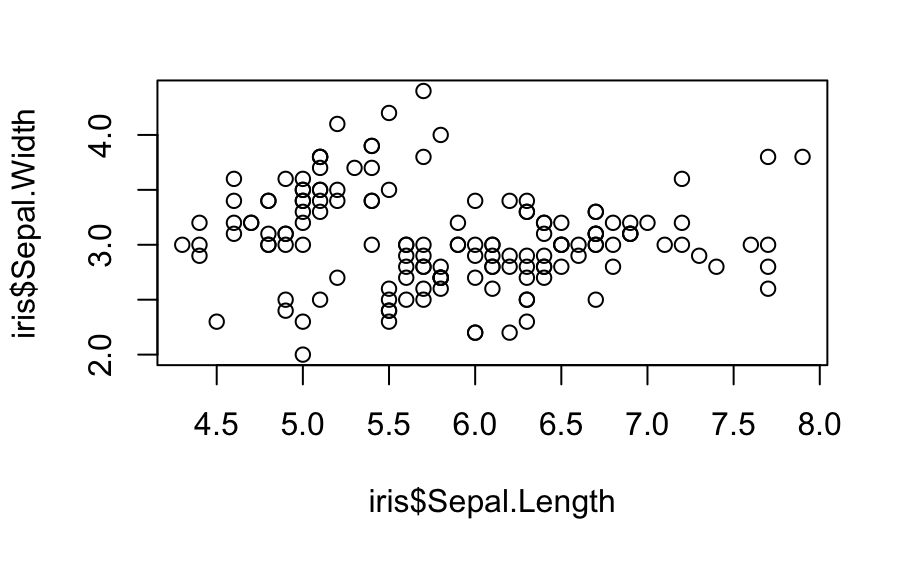The same plot in ggplot is a bit more complicated to put together:
``````ggplot(data = iris, mapping = aes(x = Sepal.Length, y = Sepal.Width)) +
geom_point()``````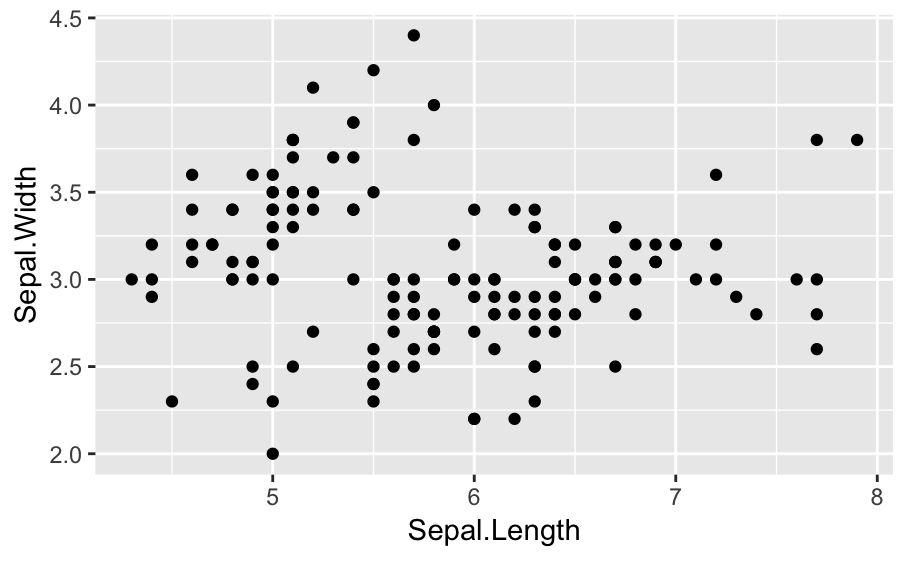The above contains the components that are the bare minimum of what we need for a ggplot plot; we can add more on later, but let’s dissect the parts of this command:
``````ggplot(data = <DATA>, mapping = aes(<Mapping>)) +
<GEOM_FUNCTION>()``````
• arguments:
• data: the dataframe you want to plot
• mapping: Any variables from your data that affect plot output, listed in aes( )
• commands:
• ggplot( ): required start of every ggplot command. Contains any options that we want to apply to the whole plot (which can be nothing)
• geom_{something}( ): how you’re plotting the data. Here, we want to plot points, so we’re using geom_point; there are tons of different geoms available, one for each type of plot you might want to make.
Arguments like data and mapping can go in the parentheses after the geom, producing the same plot as above:
``````ggplot() +
geom_point(data = iris, mapping = aes(x = Sepal.Length, y = Sepal.Width))``````But there are specific situations in which it’s better to do this (we’ll see them later)

## Modifying geom properties

We can also pass additional arguments to the geom: useful ones to know are:
• color: line color; for the default shape used in geom_point, this actually colors the inside of the shape as well
• fill: the fill color inside a shape
• size: point size or line thickness
• shape: for points, this is the shape; for lines, this is the line pattern or dashyness
• alpha: transparency level, with 0 being totally transparent and 1 being a solid, opaque color
For example:
``````ggplot(data = iris, mapping = aes(x = Sepal.Length, y = Sepal.Width)) +
geom_point(color = 'blue', fill = 'yellow', shape = 23, alpha = 0.33, size = 5)``````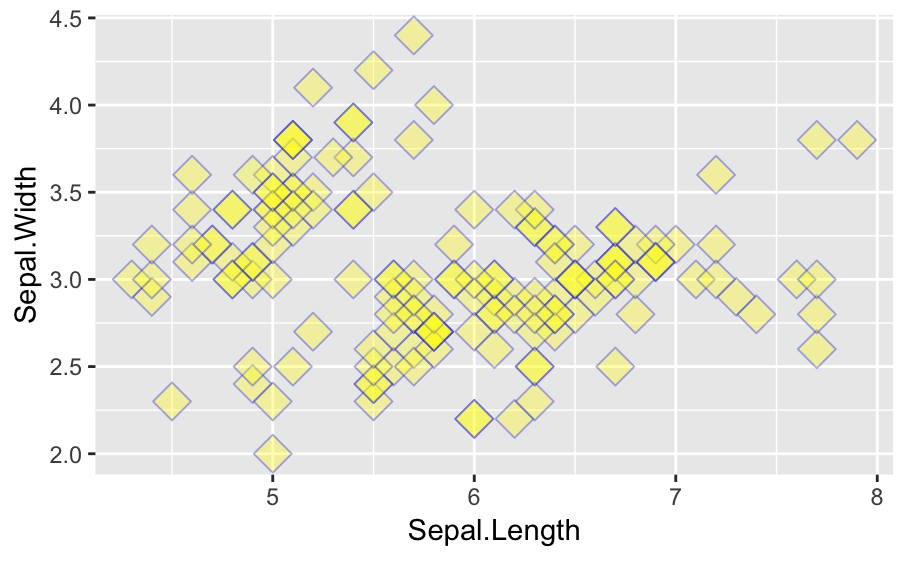Why are some of these rhombuses darker than others? Note that any arguments that universally affect the properties of the points, lines, etc that we’re plotting, like the ones we used above, must be passed to the relevant geom, not to the ggplot( ) command. This is because the geom is in charge of making the points!

## Mapping lots of variables

The plot we made above isn’t really all that useful. It’s great to see the data across all three species on one plot, but if we’re looking at this data, we’re probably actually interested in how these species differ from each other. So how do we make ggplot visually separate the points by species? Remember that the mapping argument deals with any properties of the plot that depend on variables in the supplied data frame. So we can modify our original code like this:
``````ggplot(data = iris, mapping = aes(x = Sepal.Length, y = Sepal.Width, color = Species)) +
geom_point(alpha = 0.33)``````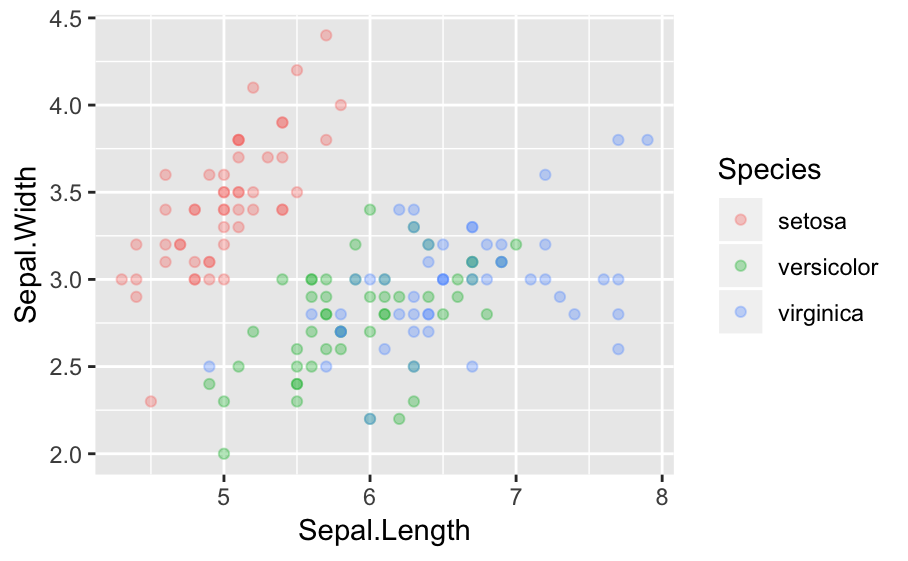``````# Can also be written as:
ggplot(data = iris) +
geom_point(mapping = aes(x = Sepal.Length, y = Sepal.Width, color = Species), alpha = 0.33)``````
Notice that the plot above uses both a variable-dependent color (based on the iris dataframe’s Species column), which goes inside aes( ), and a variable-independent alpha value that applies to the whole geom_point command and goes outside aes( ) Also, notice that you got a legend for free! You didn’t have to tell ggplot how to make it, or what info to include in it; it knows automatically based on how you set up your mapping. Depending on context, you can make color, fill, shape, size or alpha variable-dependent. Some of these (color, fill, shape) obviously make more sense for categorical variables, while others (alpha, size) make more sense for continuous variables, but ggplot will only rarely stop you from making aesthetically and data representationally questionable choices here. Let’s try an exercise: based on the code above, make a plot where Sepal.Length is on the x axis, Sepal.Width is on the y axis, all the points are colored red, the shape of the point depends on the Species, and the point size depends on Petal.Width Questionable usefulness, but hey, it’s possible and pretty easy…

## Stacking multiple geoms

One of the places where ggplot really shines is when you want to combine multiple data representations on one plot. For example, I really like topology-style contour plots, which ggplot can make with geom_density2d. Once we know how to make a basic plot, and combining a contour plot with a plot the individual data points is super easy in ggplot:
``````# note, the first two lines are just our plot from above
ggplot(data = iris, mapping = aes(x = Sepal.Length, y = Sepal.Width, color = Species)) +
geom_density2d() +
geom_point(alpha = 0.33)``````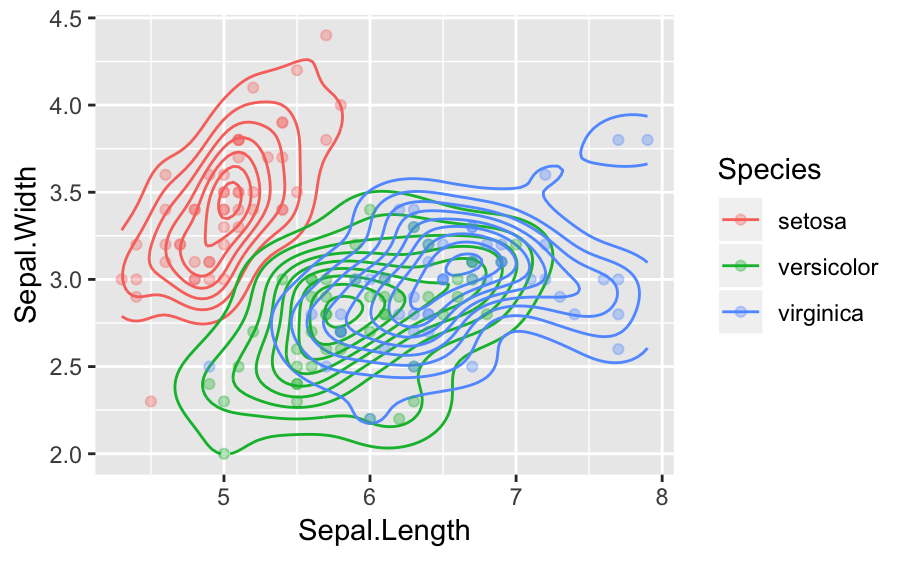Notice that the alpha argument we provided only applies to geom_point, so the contour lines don’t show any transparency. However, any arguments provided to mapping in an aes( ) statement in the ggplot( ) command apply across all geoms. (Also, notice that when we add a geom, ggplot automatically updates our legend!) One really powerful application of this is that we can actually make each geom( ) represent a different aspect of the same data. Let’s say we’d like our datapoints to be colored by species, but we’d also like to see a contour plot of sepal length vs width across all the species. To do this, we’re going to have to move our mapping calls inside the geoms, since we now want each geom to map the data differently:
``````# got rid of alpha here just to simplify things
ggplot(data = iris) +
geom_density2d(aes(x = Sepal.Length, y = Sepal.Width)) +
geom_point(aes(x = Sepal.Length, y = Sepal.Width, color = Species))``````